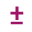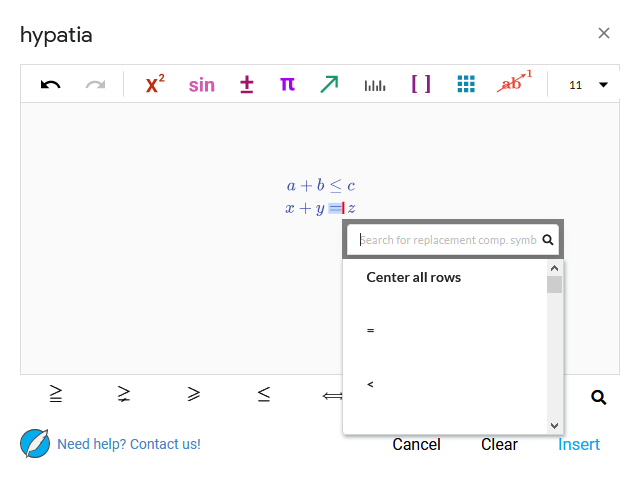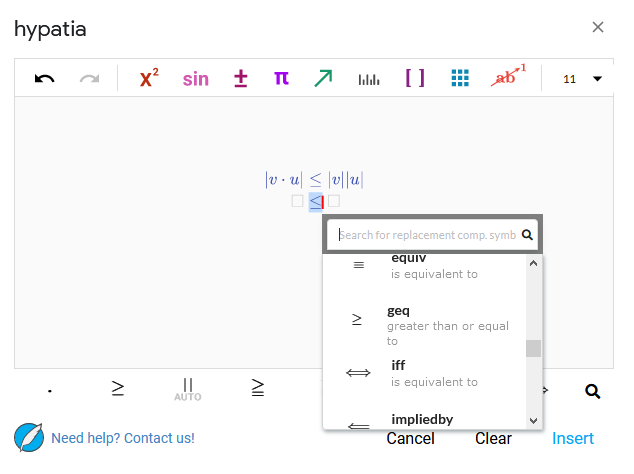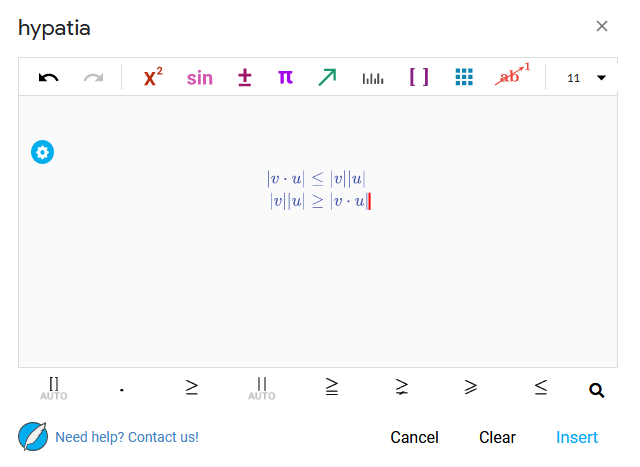# How can you change the equality sign in math equations?

When typing a single line of math in the hypatia editor, you can simply choose which relation symbol you want to add using the Binary Operators menu () and insert it as you type. This menu is shown in the figure below.In multiline math however, the relation symbol is automatically inserted when a new line is created. To replace the symbol that is currently there, set your text cursor to the position just after the symbol and press the `Back Space` key. This will bring up a menu of all possible relation symbols as shown in figure below. Selecting a symbol from this menu will change the symbol on the current line.Each new line created will automatically use the relation symbol from the previous line.

# Example

As an example, let’s write out the Cauchy-Schwartz inequality on one line and then write it again but with the sides switched below.

Let’s start by opening the full editor and typing out the inequality as shown in the figure below.Next, let’s position the text cursor at the end of the equation and hit the `Enter` key to create a multiline math environment. Let’s choose ‘Aligned Multiline Equation’ and move the text cursor to the right side. Let’s now hit the `Back Space` key to bring up the relations menu and choose the greater than or equal to relation as shown in the figure below.Now that we have the opposite relation symbol, fill in both sides and we’re done. The finished multiline math is shown below.